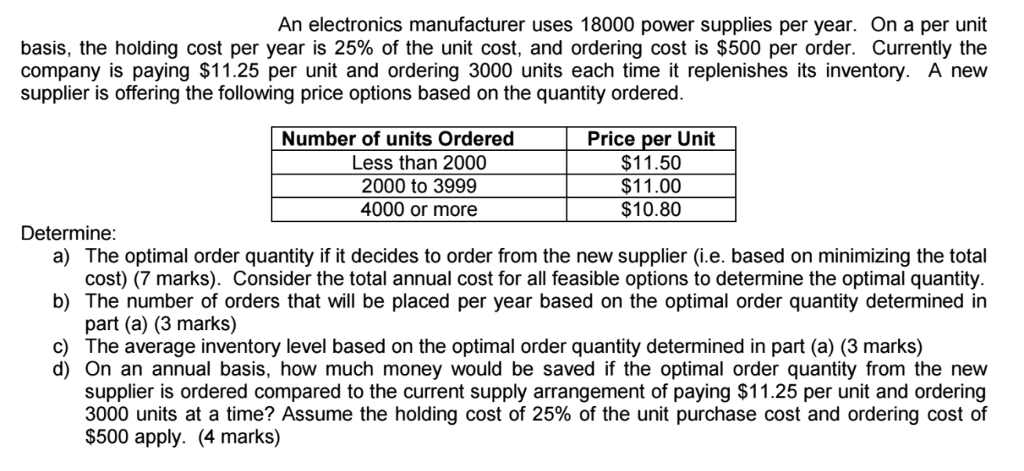# Solved: SHOW ALL STEPS FOR THE QUESTION TO BE WORTH ANY MARKS. SHOW FORMULAS

SHOW ALL STEPS FOR THE QUESTION TO BE WORTH ANY MARKS. SHOW FORMULAS WHEN GETTING NUMBERS and MAKE IT CLEAR HOW YOU ARE ARRIVING IN THE NUMBERS.Don't use plagiarized sources. Get Your Custom Essay on
Solved: SHOW ALL STEPS FOR THE QUESTION TO BE WORTH ANY MARKS. SHOW FORMULAS
GET AN ESSAY WRITTEN FOR YOU FROM AS LOW AS \$13/PAGE

An electronics manufacturer uses 18000 power supplies per year. On a per unit basis, the holding cost per year is 25% of the unit cost, and ordering cost is \$500 per order. Currently the company is paying \$11.25 per unit and ordering 3000 units each time it replenishes its inventory. A new supplier is offering the following price options based on the quantity ordered. Number of units Ordered Less than 2000 2000 to 3999 4000 or more Price per Unit \$11.50 \$11.00 \$10.80 Determine a) The optimal order quantity if it decides to order from the new supplier (i.e. based on minimizing the total b) The number of orders that will be placed per year based on the optimal order quantity determined in c) cost) (7 marks). Consider the total annual cost for all feasible options to determine the optimal quantity part (a) (3 marks) The average inventory level based on the optimal order quantity determined in part (a) (3 marks) d) On an annual basis, how much money would be saved if the optimal order quantity from the new supplier is ordered compared to the current supply arrangement of paying \$11.25 per unit and ordering 3000 units at a time? Assume the holding cost of 25% of the unit purchase cost and ordering cost of \$500 apply. (4 marks)

Given Demand D = 18000

Ordering cost S = \$500

Old supplier:

Cost C = \$11.25

Holding cost H = 25% = 0.25*11.25 = 2.8125

New supplier:

 Range Price C Holding cost Less than 2000 11.50 2.875 2000-3999 11.00 2.75 4000 or more 10.80 2.70

a) We know Economic order quantity (EOQ)  Q

We will calculate EOQ at different price levels

At C = \$11.50

Q = 2502.17

It is not feasible as it is not in the range of below 2,000

At C = 11

Q = 2558.41

It is feasible as it is with in the range of 2,000 – 3,999

At C = 10.80

Q = 2581.99 or 2582

It is feasible as it is not in the range of above 4000

At Q = 2558 is feasible but we are getting more discount at more than 4000 or above. So we calcualte total cost at Q = 2558 and 4000

Total cost TC = Purchase cost + Annual holding cost + annual ordering cost

TC = (C*D) + (Q/2) H+ (D/Q) S

At Q = 2558

TC = (11*18000) + (2558/2)*2.75 + ( 18000/2558)*500 = 198000 + 3517.25 + 3518.37 = 205035.62

At Q = 4000

TC = (10.8*18000) + (4000/2)*2.7 + ( 18000/4000)*500 = 194400 + 5400 + 2250 = 202050

Total cost is less at Q = 4000 units

Optimal quantity Q = 4000 units

b) No of orders = (D/Q) = 18000/4000 = 4.5 orders or 5 orders

c) Average inventory level = Q/2 = 4000/2 =2000

d) Total cost at Q = 3000 units and H = 2.8125

TC = (11.25*18000) +(3000/2)*2.8125 +(18000/3000)*500 = 202500 + 4218.75 + 3000 = 209718.75

Savings = 209718.75 – 202050 = 7668.75